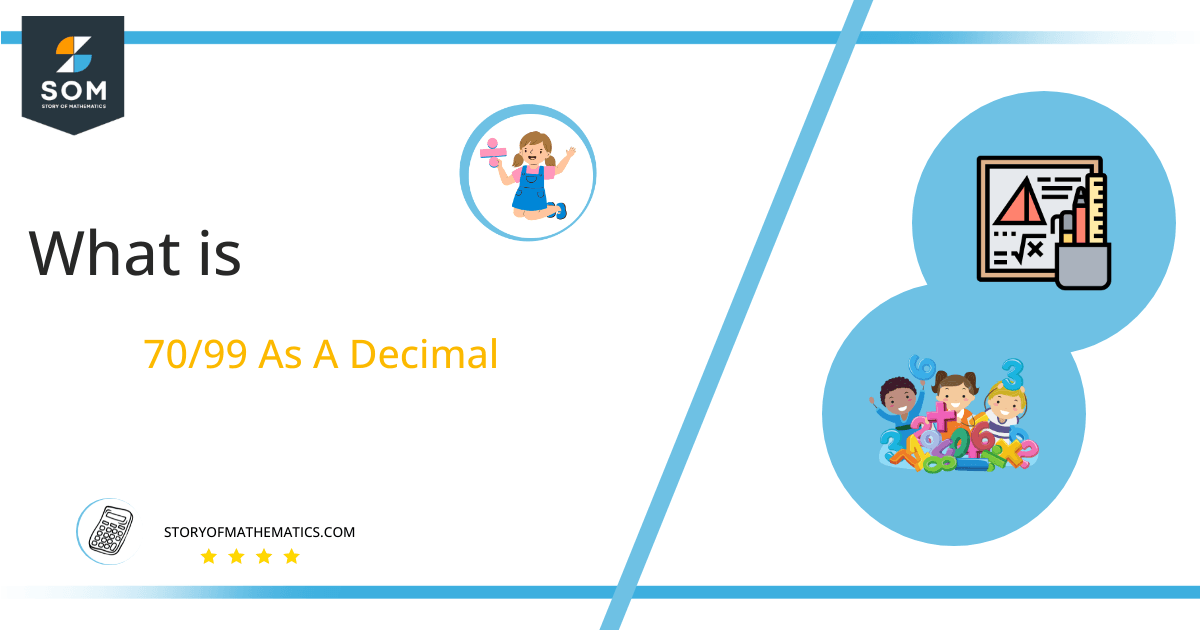# What Is 70/99 as a Decimal + Solution With Free Steps

The fraction 70/99 as a decimal is equal to 0.707.

The division of two numbers p and q produces either an integer result or a decimal value (that may or may not terminate). If the dividend is both greater than and a multiple of the divisor, we get the former result. Otherwise, we get the latter result.Here, we are more interested in the division types that result in a Decimal value, as this can be expressed as a Fraction. We see fractions as a way of showing two numbers having the operation of Division between them that result in a value that lies between two Integers.

Now, we introduce the method used to solve said fraction to decimal conversion, called Long Division, which we will discuss in detail moving forward. So, let’s go through the Solution of fraction 70/99.

## Solution

First, we convert the fraction components, i.e., the numerator and the denominator, and transform them into the division constituents, i.e., the Dividend and the Divisor, respectively.

This can be done as follows:

Dividend = 70

Divisor = 99

Now, we introduce the most important quantity in our division process: the Quotient. The value represents the Solution to our division and can be expressed as having the following relationship with the Division constituents:

Quotient = Dividend $\div$ Divisor = 70 $\div$ 99

This is when we go through the Long Division solution to our problem.Figure 1

## 70/99 Long Division Method

We start solving a problem using the Long Division Method by first taking apart the division’s components and comparing them. As we have 70 and 99, we can see how 70 is Smaller than 99, and to solve this division, we require that 70 be Bigger than 99.

This is done by multiplying the dividend by 10 and checking whether it is bigger than the divisor or not. If so, we calculate the Multiple of the divisor closest to the dividend and subtract it from the Dividend. This produces the Remainder, which we then use as the dividend later.

Now, we begin solving for our dividend 70, which after getting multiplied by 10 becomes 700.

We take this 700 and divide it by 99; this can be done as follows:

700 $\div$ 99 $\approx$ 7

Where:

99 x 7 = 693

This will lead to the generation of a Remainder equal to 700 – 693 = r1. Now this means we have to repeat the process by Converting the r1 into 700. Doing so requires a double multiplication by 10 since 7 x 10 = 70 is smaller than 99. Thus, we add a 0 to our quotient for the second multiplication. Solving for that:

700 $\div$ 99 $\approx$ 7

Where:

99 x 7 = 693

Finally, we have a Quotient generated after combining the three pieces of it as 0.707, with a Remainder equal to 7.

Images/mathematical drawings are created with GeoGebra.